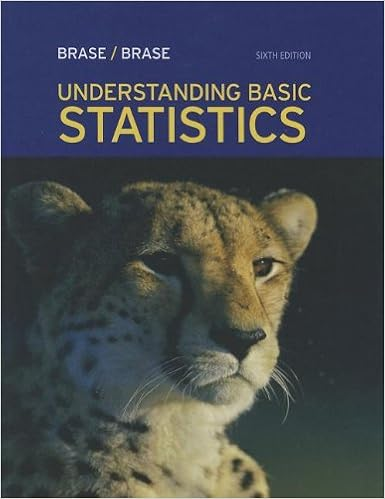# 2 compare the width of the lines on the left and

• Test Prep
• ayyyy1995
• 9
• 100% (3) 3 out of 3 people found this document helpful

This preview shows page 7 - 9 out of 9 pages.

##### We have textbook solutions for you!
The document you are viewing contains questions related to this textbook.The document you are viewing contains questions related to this textbook.
Chapter 11 / Exercise 2
Understanding Basic Statistics
Brase/BraseExpert Verified
2. Compare the width of the lines on the left and right side. The right side is much wider. Compare the sides to the left and right of the vertical line inside the box. The right side is much wider. Both facts are consistent with data that is skewed to the right.3. A score of 400 is the 25’th percentile. So, approximately 25% of the scores are below 400.4. Needed numbers are the minimum, 25’th percentile, median, 75’th percentile, and maximum.5. These are values within two standard deviations of the mean. That’s from 510 – 2(100) to 510 + 2(100) or from 310 to 710.6. That’s the 4 leaves that go with scores in the 80’s or 90’s.7. The cumulative relative frequency is the sum of the relative frequencies for the first five classes. This is .03 + .07 + .15 + .15 +.25 = .65.8. Add the frequencies for the first three classes. This is 8 + 19 + 15 = 42.9. See the chapter 2 handout10. Approximately 50% of the values are above or below the median11. Use the formula for computing S. The sample mean is 4. The deviations from the mean are 1, -2, -3, 4. The square of these values are 1, 4, 9, 16. The sum of these is 30. The standard deviation is square root of 30/(4-1) = 3.16.12. See chapter 3 handout about SHAPE of a set of data.13. Calculate x P(x) for each value of x and then sum. The result is 0 + .2 + .2 + .3 = .7.14. Less than or equal to 2 includes X =2 or 1 or 0. Add the probabilities for these values of x. The result is .1 + .2 + .6 = .915. More than 1 includes X =2 or 3. Add the probabilities for these values of x. The result is .1 + .1 = .2
##### We have textbook solutions for you!
The document you are viewing contains questions related to this textbook.The document you are viewing contains questions related to this textbook.
Chapter 11 / Exercise 2
Understanding Basic Statistics
Brase/BraseExpert Verified
16. The mean of a probability distribution is interpreted as a “long term average”. In this case, that would mean the average number of defects if X was observed a large number of times. But that means the average number when we observe a large number of these products.17. The total for the one vehicle column.18. The intersection of the labels “one vehicle” and “Yes”.19. P(one vehicle | yes) = .125 / .425 = .294.PROBLEMS1 a) .855b) Use addition rule: P(first works or second works) = P(first works) + P(second works) – P(first works and second works) = .95 + .90 - .855 = .995c) I’ll use A for “first system fails” and B for “second system fails”. It turns out that A and B are independent events. This can be justified using probabilities in any of three ways. These are by showing thatP(A and B) = P(A) P(B) or by showing P(A) = P(A|B) or by showing P(B) = P(B|A).Here are the numbers: P(A) = .05, P(B) = .10, P(A and B) = .005, P(A|B) = .005/.10 = .05,P(B|A) = .005/.05 = .10.2.First set up a probability table.
•••